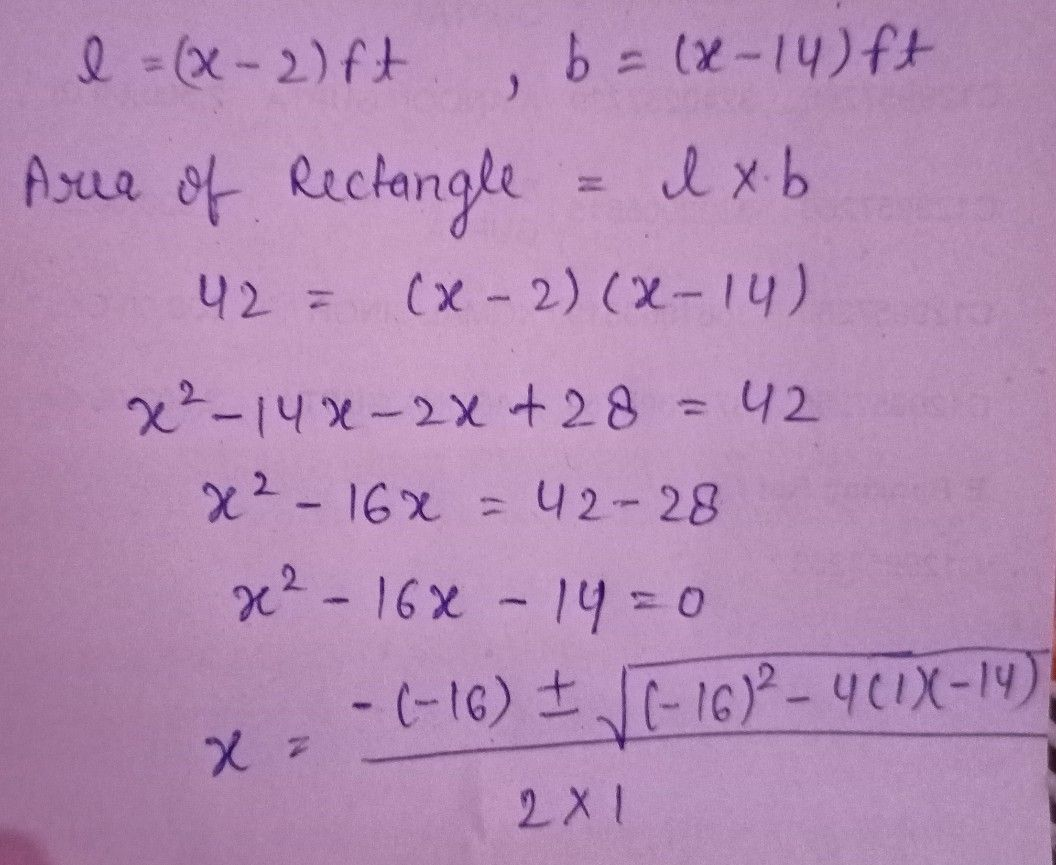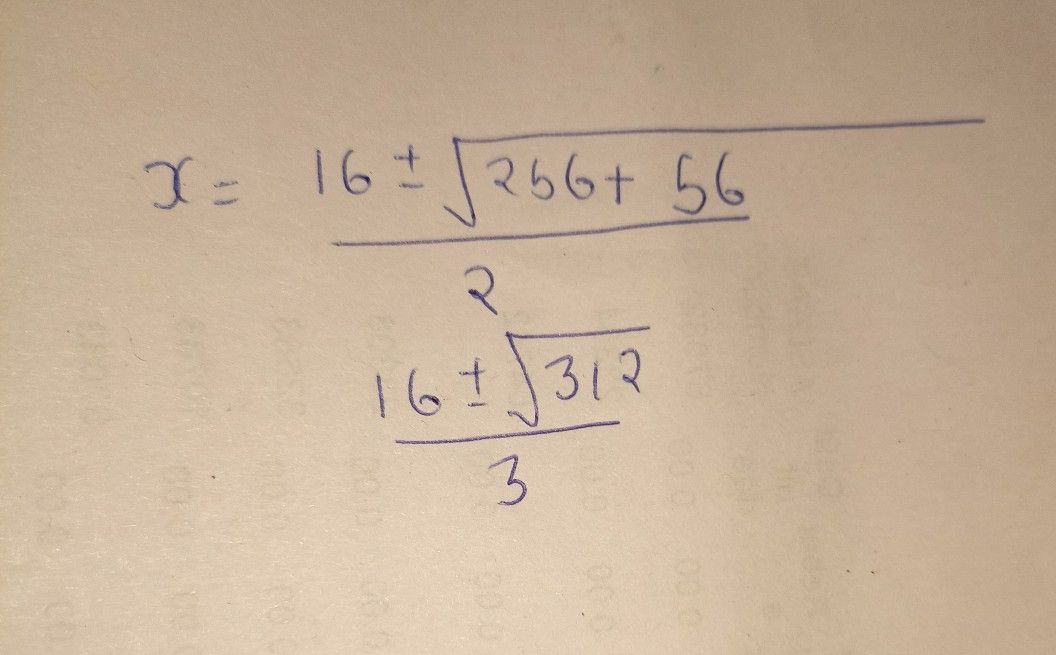Symbol
ProblemIn Exercises $11$ find the dimensions of the polygon with the given area. $11$ Area $ea=42sq$ feet $\left(x-14\right)+$ $\bar{\left(x-2\right)}$ $\pi$